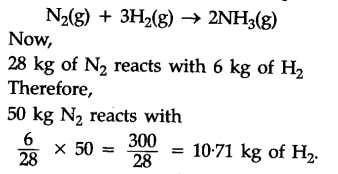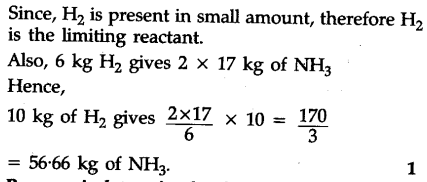# 50.0 kg of \$N_{2}\$(g) and 10.0 kg of \$H_{2}\$ (g) are mixed to produced N\$H_{3}\$ (g). Calculate the N\$H_{3}\$(g) formed

50.0 kg of \$N_{2}\$(g) and 10.0 kg of \$H_{2}\$ (g) are mixed to | produced N\$H_{3}\$ (g). Calculate the N\$H_{3}\$(g) formed. Identify the limiting reagent in the production of N\$H_{3}\$ in this situation